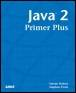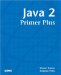# Chapter 3Java™ 2 Primer PlusBy Steven Haines, Steve Potts Table of Contents Appendix C.  Answers to Review Questions

 A1: The modulus operator (%) returns the remainder of integer division:10 % 3 = 1, or explicitly 10 / 3 = 3 with remainder 1 A2: The && operator is the logical AND and is used in Boolean operations that compare two values, for example: ` if( a > 5 && a < 10 ) ... `This will evaluate to true only with a value between 5 and 10.The & operator is the bitwise AND and is used when performing the bitwise AND of type numbers. For example:3 & 9 = 1, or explicitly 0000 0011 & 0000 1001 = 0000 0001 A3: A truth table is a list of all possible input values and their corresponding values. For example the truth table for the AND operation isA B Result0 0 00 1 01 0 01 1 1 A4: 21 & 3 == 0001 0101 & 0000 0011 = 0000 0001 = 1 (AND) A5: 21 | 3 == 0001 0101 | 0000 0011 = 0001 0111 = 23 (OR) A6: 21 ^ 3 = 0001 0101 ^ 0000 0011 = 0001 0110 = 22 (XOR) A7: >> is right shift while >>> is right shift fill with 0s. >> fills with the left most bit. A8: 5 * 5 + 5 is equivalent to ( 5 * 5 ) + 5 = 25 + 5 = 30 A9: 5 + 5 * 5 is equivalent to 5 + ( 5 * 5 ) = 5 + 25 = 30 A10: Evaluate the following: ` int a = 5; a += 6 * ++a / 2 6 * 9 + 2 a += ( ( ( 6 * ++5 ) / 2 ) (6 * 9) ) + 2 = a += ( ( ( 6 * 6 ) / 2 ) (6 * 9) ) + 2 = a += ( ( ( 36 ) / 2 ) (54) ) + 2 = a += ( 18 54 ) + 2 = a += ( 36 ) + 2 = a += -34 = a = a 34 = a = 5 34 = a = -29 `

 TopJava 2 Primer Plus
ISBN: 0672324156
EAN: 2147483647
Year: 2001
Pages: 332

Similar book on Amazon